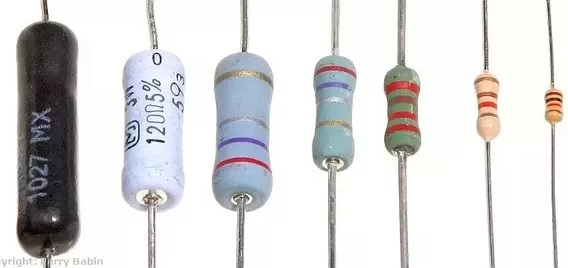# How can we control voltage using resistor?Within a circuit it is often necessary to have different voltages at different stages and we can achieve this by using resistors. For example, if we physically opened up a resistor and connected its ends across a supply, we would find that, if we then measured the voltage at different points along the resistor, the values would vary along its length.lets discuss about How can we control voltage using resistor?

However, reality will stop us from doing this, as resistors are sealed components. But we can create the same tapping effect by combining two resistors in series as shown in Figure , and then our tapping becomes a connection point made between the two resistors.If we look at Figure , we can see that the series combination of resistors R1 and R2 is connected across a supply that is provided by two rails. One is shown as V + (the positive supply rail or, in other words, our input) and the other as 0 V (or common rail of the circuit).

The total resistance of our network (Rt) will be: Rt = R1 + R2
We know, using Ohm’s law, that V = I × R and the same current flows through both resistors. Therefore, for this network, we can see that V+= I × Rt, and the voltage dropped across resistor R2, Vo= I × R2.

We now have two expressions, one for V+ and one for Vo. We can find out what fraction Vo is of V+ by putting Vo over V+ on the left-hand side of an equation and then putting what we said each one is equal to in the corresponding positions on the right-hand side. This gives us the following formula:As current is common on the right hand side of our formula, they cancel each other out. This leaves us with:To establish what Vo (our output voltage) actually is, we can transpose again, which would give us:Finally, we can replace Rt by what it is actually equal to, and this will give us the means of establishing the value of the individual resistors needed to give a desired output voltage (Vo). By transposition, our final formula now becomes:This equation is normally referred to as the potential divider rule.

In reality R1 and R2 could each be a combination (series or parallel) of many resistors. However, as long as each combination is replaced by its equivalent resistance so that the simplified circuit looks like Figure then the potential divider rule can be applied.The potential divider circuit is very useful where the full voltage available is not required at some point in a circuit and, as we have seen, by a suitable choice of resistors in the potential divider, the desired fraction of the input voltage can be produced.

In applications where the fraction produced needs to be varied from time to time, the two resistors are replaced by a variable resistor (also known as a potentiometer, which is often abbreviated to the word pot), which would be connected as shown in Figure.

The potentiometer has a resistor manufactured in the form of a track, the ends of which effectively form our V+ and 0 V connections. Our output voltage (Vo) is achieved by means of a movable contact that can touch the track anywhere on its length and this is called the wiper. We have therefore effectively created a variable tapping point.
To compare this with our potential divider, we can say that the part of the track above the wiper can be regarded as R1 and that part below the wiper as R2. The fraction of the input voltage appearing at the output can therefore be calculated for any setting of the wiper position by using our potential divider equation:Obviously, when the wiper is at the top of the track, R1 becomes zero and the equation would give the result that Vo is equal to V+. Equally, with the wiper right at the bottom of the track, R2 now becomes zero and therefore Vo also becomes zero, which is not too surprising as the wiper is now more or less directly connected to the 0 V rail. This sort of circuit finds practical application in a wide variety of control functions, such as volume or tone controls on audio equipment, brightness and contrast controls on televisions and shift controls on oscilloscopes.